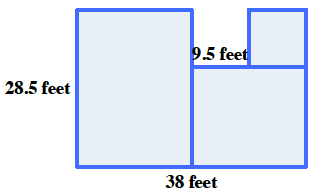### Home > ACC6 > Chapter 8 Unit 8 > Lesson CC1: 8.1.5 > Problem8-69

8-69.

Draw a figure that is made of rectangles and has a perimeter of $152$ feet. Then show how to find its area.

Remember that the perimeter is the distance around a shape and the area is the space within the shape.
Below is one example of a figure with a perimeter of 152 feet. Can you draw a different figure?